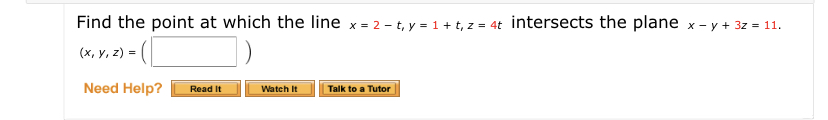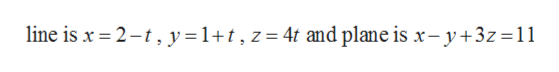# Find the point at which the line x = 2 – t, y = 1+ t, z = 4t intersects the plane x - y + 3z = 11.(x, y, z) = (|Need Help?Watch ItTalk to a TutorRead It

Question
1 viewshelp_outlineImage TranscriptioncloseFind the point at which the line x = 2 – t, y = 1+ t, z = 4t intersects the plane x - y + 3z = 11. (x, y, z) = (| Need Help? Watch It Talk to a Tutor Read It fullscreen
check_circle

Step 1

Given,

...help_outlineImage Transcriptioncloseline is x = 2-t, y=1+t, z= 4t and plane is x – y +3z=11 fullscreen

### Want to see the full answer?

See Solution

#### Want to see this answer and more?

Solutions are written by subject experts who are available 24/7. Questions are typically answered within 1 hour.*

See Solution
*Response times may vary by subject and question.
Tagged in

### Other